### Home > CC1 > Chapter 7 > Lesson 7.3.1 > Problem7-86

7-86.
1. Recall that inverse operations “undo” each other. Write the inverse operation for each situation below. Homework Help ✎

1. Add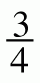.

2. Subtract 1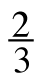.

3. Divide by 8.

4. Multiply by 12.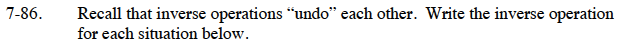Addition and subtraction are inverse operations.
For example, adding 4 and then subtracting 4 takes you back to your original number.

Multiplication and divsion are also inverse operations.
For example, multiplying a number by 4 and then dividing by 4 takes you back to your original number.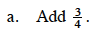$\text{Subtract}\ \frac{3}{4}.$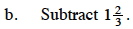If subtraction and addition are inverse operations, how much do you have to add?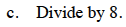Multiply by 8.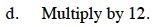If multiplication and division are inverse operations, how much do you have to divide by?